Latest Banking jobs   »   Reasoning Ability Quiz For SBI Clerk...

# Reasoning Ability Quiz For SBI Clerk Mains 2022- 27th December

Directions (1-5): Answer the questions based on the information given below. In every question two rows are given and to find out the resultant of the particular row you need to follow the following steps:
Step 1: If an odd number is followed by an odd number (not prime number), then the resultant will be the smaller of two numbers.
Step 2: If an odd number is followed by an odd/even prime number, then the resultant will be the product of two numbers.
Step 3: If an even number is followed by an even number, then the resultant will be the absolute difference of two numbers.
Step 4: If an odd number is followed by an even number, then the resultant will be the addition of two numbers.
Step 5: If an even number is followed by an odd number, then the resultant will be the remainder, when the greater number is divided by the smaller number.

Q1. What is the addition of the resultant of both the rows?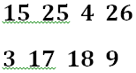(a) 51
(b) 50
(c) 54
(d) 56
(e) None of these

Q2. Which of the following combination of numbers represents the resultant of Row I and Row 2, respectively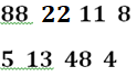(a) 2, 117
(b) 116, 2
(c) 2, 115
(d) 4, 116
(e) None of these

Q3. What is the resultant of Row 2 if K is the resultant of Row 1?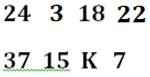(a) 188
(b) 189
(c) 190
(d) 191
(e) None of these

Q4. What is the multiplication of the resultant of both the rows?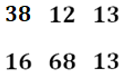(a) 10
(b) 16
(c) 18
(d) 8
(e) None of these

Q5. What is the difference of the resultant of both the rows?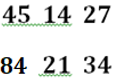(a) 5
(b) 3
(c) 4
(d) 2
(e) None of these

Q6. Choose the option which shows the group of letters which will complete the given sequence.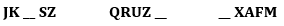(a) O, F, V
(b) M, H, U
(c) N, F, W
(d) N, G, W
(e) None of these

Q7. How many pairs of letters are there in the word ‘PENNSYLVANIA’ which has as many letters between them as in English alphabetical series?
(a) Three
(b) Four
(c) Five
(d) Two
(e) None of the above

Q8. If 3rd letter from the right end of each word from each group is to be considered, then which among the following group of words can make at least one 4-letter meaningful English word such that one letter to be used only once?
II. ROCK, MATE, STEM, MOOT
III. DEAL SEIP, LIVE, MORE
(a) Only I and III
(b) Only I
(c) Only II
(d) Only II and III
(e) None

Q9. If all the letters which appear more than once in the word ‘CONJUNCTIVELY’ are dropped then how many such pair(s) of letters are there in the newly formed word which has as many letters between them as in the alphabetical series?
(a) Three
(b) One
(c) Four
(d) More than four
(e) None of these

Q10. If all the vowels of the word ‘MORNING’ are replaced by its succeeding letter according to the English alphabet and all the consonants are replaced with their previous letter according to the English alphabet. Then all the letters are arranged in the alphabetical order the how many letters are there between the third letter from the left and second letter from the right in the English alphabetic series?
(a) Three
(b) Five
(c) Four
(d) More than five
(e) None of these

Q11. If in ‘3687156492′ each digit at the odd place from the left end remain unchanged while each digit at the even place from the left end is increased by one, then how many such pairs of the digits are there in the number thus formed, which have as many digits in the number as they have been arranged in the descending and ascending order?
(a) Three
(b) Six
(c) Four
(d) Seven
(e) None of these

Q12. The position of first and twelfth letters in the word ‘JEOPARDIZING’ are interchanged. Similarly, the positions of second and eleventh letters are Interchanged and the position of third and tenth letters are interchanged and so on, then which of the following alphabet is the immediate succeeding alphabet as per English alphabetical order of eighth alphabet from the right end in the new word?
(a) B
(b) J
(c) A
(d) S
(e) None of these

Q13. If 1 is subtracted from each odd digit and 1 is added to each even digit in the two numbers given in two groups I and II given below then arrange each digit of each group is ascending order from right to left, then what is the sum of the digits which are 5 from the left end in both the groups?
I. 8459763
II. 67298357
(a) 11
(b) 10
(c) 12
(d) 16
(e) None of these

Q14. How many pairs of letters are there in the word ‘DRAWBACK’ which has as many letters between them as in English alphabetical series?
(a) Three
(b) Four
(c) Five
(d) Two
(e) None of the above

Q15. How many pairs of letters are there in the word ‘SPLINTER’ which has as many letters between them as in English alphabetical series?
(a) Three
(b) Four
(c) Five
(d) Two
(e) None of the above

Solutions

S1. Ans. (c)
Sol. In row 1, according to the given step, resultant of row 1 is 45.
In row 2, according to the given step, resultant of row 2 is 9.
Hence, required sum = 45 + 9 = 54.

S2. Ans. (a)
Sol. In row 1, according to the given step, resultant of row 1 is 2.
In row 2, according to the given step, resultant of row 2 is 117.

S3. Ans. (b)
Sol. In row 1, according to the given step, resultant of row 1 is 12. So, K = 12.
In row 2, according to the given step, resultant of row 2 is 189.

S4. Ans. (d)
Sol. In row 1, according to the given step, resultant of row 1 is 2.
In row 2, according to the given step, resultant of row 2 is 4.
Hence, required multiplication = 2 x 4 = 8.

S5. Ans. (b)
Sol. In row 1, according to the given step, resultant of row 1 is 27.
In row 2, according to the given step, resultant of row 2 is 30.
Hence, required difference = 30 – 27 = 3.

S6. Ans. (d)
Sol.
J K (LM) N (OPQR) S (TUVWXY) Z
Q R (ST) U (VWXY) Z (ABCDEF) G
W X (YZ) A (BCDE) F (GHIJKL) M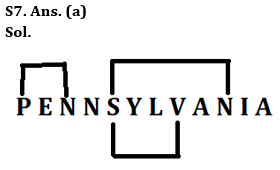S8. Ans. (e)
Sol.
I. READ, TEAM, POOR, SALE – The 3rd letter of given words from the right end are ‘E, E, O, and A. No meaningful English word can be formed.
II. ROCK, MATE, STEM, MOOT – The 3rd letter of given words from the right end are ‘O, A, T, and O. No meaningful English word can be formed.
III. DEAL, SEIP LIVE, MORE – The 3rd letter of given words from the right end are ‘E, E, I, and O’. No meaningful English word can be formed.

S9. Ans. (d)
Sol. The given word – CONJUNCTIVELY
After dropping the letters ‘C’ & ‘N’ the new word is ‘OJUTIVELY’.
There are six such pairs i.e., IL, EJ, TY, TU, VY and TV, which have as many letters between them as in the alphabetical series.

S10. Ans. (a)
Sol. The given word – MORNING
After interchanging the vowels and consonants of the given word – LPQMJMF
Now, rearranging all the letters according to the English alphabetical series – FJLMMPQ
Hence, there are three number of letters between the third letter from the left i.e. ‘L’ and second letter from the right i.e., “P” in the English alphabetic series.

S11. Ans. (b)
Sol. Given number: 3687156492
After rearrangement we get: 3788166593
So, there are 6 such pairs i.e., 78, 68, 69, 56, 35 and 36.

S12. Ans. (b)
Sol. Given word: JEOPARDIZING
After interchanging the letters, we get the new word i.e., GNIZIDRAPOEJ
So, the 8th letter from the right end is I and the immediate succeeding alphabet of I is J.

S13. Ans. (a)
Sol. After operation –
I. 8459763 – 9548672 – 9876542
II. 67298357 – 76389246 – 98766432
So, the sum of the digits which are 5th from the left end in both the groups are 5 and 6 i.e., 11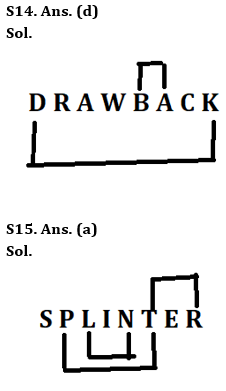#### Congratulations!Union Budget 2023-24: Free PDF